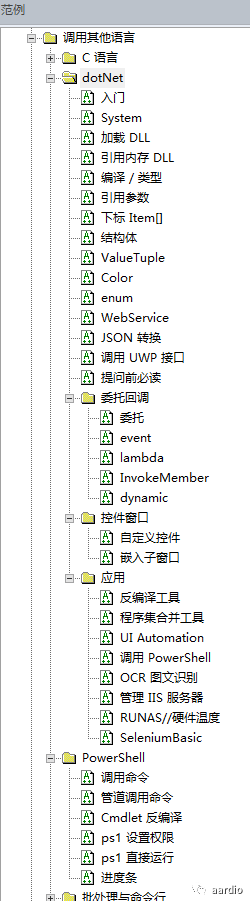# aardio + C# 重大更新来了！

``[MarshalAs(UnmanagedType.ByValTStr, SizeConst = 20)] ``

``````import dotNet;
var compiler = dotNet.createCompiler("C#");
compiler.Source = /***
namespace CSharpLibrary
{
public struct TestStruct
{
public TestStruct(string n, double v)
{
Name = n;
Value = v;
}

public string Name;
public double Value;
};
}
***/

//编译生成程序集，导入 C# 名字空间
var CSharpLibrary = compiler.import("CSharpLibrary");

//调用 C# 创建结构体
var testStruct = CSharpLibrary.TestStruct("测试",12345);

//输出值看一下
import console;
console.logPause(testStruct.Name,testStruct.Value);``````

``````namespace TestValueTuple
{
public class Class1
{
public static (double, int, int, int, int, int, int, int, int, int, int) tupleValue = (12.3, 22, 22, 22, 22, 22, 22, 22, 22, 22, 22);
public static  (double, int) GetTupleValue()
{
return (12.3, 22);
}
}
}``````

``````import console;
import dotNet;

//加载程序集 TestValueTuple.dll 并导入 TestValueTuple 名字空间
var TestValueTuple = dotNet.import("TestValueTuple");

//直接获取 C# 中的 tuple
var tuple = TestValueTuple.Class1.tupleValue;

console.log(tuple) //调用 tostring(tuple)
console.log(tuple.Item1) //返回第 1 个元素的值
console.log(tuple.Item2) //返回第 2 个元素的值
console.log(tuple.Item7) //返回第 7 个元素的值
。
console.log(tuple.Rest) //调用 tostring(tuple.Rest)
console.log(tuple.Rest.Item1) //返回第 8 个元素的值

//当然也可以这样修改值。
tuple.Rest.Item1 = 99;
console.pause();``````

tuple 最多只能有 7 个元素，你说你想要 8 个？首先写这么长的 tuple 不至于吧？！如果真要写第 8 个，.Net 还给了个机会 —— 可以在 Rest 里放下一个 tuple 来存放更多的元素。

``````dotNet.object(value,byRef)
dotNet.byte(value,byRef)
dotNet.ubyte(value,byRef)
dotNet.word(value,byRef)
dotNet.uword(value,byRef)
dotNet.int(value,byRef)
dotNet.uint(value,byRef)
dotNet.long(value,byRef)
dotNet.ulong(value,byRef)
dotNet.float(value,byRef)
dotNet.double(value,byRef)``````

value 的值可以是普通的值或数值，也可以是数组，例如

dotNet.double({1,2,3})

``````//引用参数
import dotNet;
var compiler = dotNet.createCompiler("C#");//创建C#语言编译器
compiler.Source = /***
namespace CSharpLibrary
{
public class Object
{
public static void Test(ref double num,int [] arr){
num = 12.3;
arr = 56;
}
}
}
***/

var CSharpLibrary = compiler.import("CSharpLibrary"); //自程序集导入名字空间

//参数@2 指定 true 创建引用参数。
var num = dotNet.double(12.5,true);

//也可以创建 .Net 数组，数组总是传引用的，参数@2不必要指定为 true
var arr = dotNet.int({1,2,3});

//调用函数
CSharpLibrary.Object.Test(num,arr);

import console;
console.log( arr ) //在 aardio 中这种数组可用下标直接读写数组成员。

//如果 num 是 Primitive 类型（数值、布尔值），则可以简写为 num
console.log( num ); //等价于 dotNet.unWrapObject(num).Value

//支持 tostring(),tonumber() 转换。
console.log(tostring(num),tonumber(num));

console.pause();``````